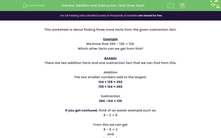# Addition and Subtraction: Find Three Facts

In this worksheet, students find three more facts from a given subtraction fact.Key stage:  KS 2

Curriculum topic:   Number: Addition and Subtraction

Curriculum subtopic:   Add/Subtract up to Four-Digit Numbers

Difficulty level:#### Worksheet Overview

This worksheet is about finding three more facts from the given subtraction fact.

Example

We know that 260 - 136 = 124.

Which other facts can we get from this?

There are two addition facts and one subtraction fact that we can find from this.

The two smaller numbers add to the largest.

124 + 136 = 260

136 + 124 = 260

Subtraction

260 -124 = 136

If you get confused, think of an easier example such as

8 - 2 = 6

From this we can get

8 - 6 = 2

and

2 + 6 = 8

6 + 2 = 8

### What is EdPlace?

We're your National Curriculum aligned online education content provider helping each child succeed in English, maths and science from year 1 to GCSE. With an EdPlace account you’ll be able to track and measure progress, helping each child achieve their best. We build confidence and attainment by personalising each child’s learning at a level that suits them.

Get started